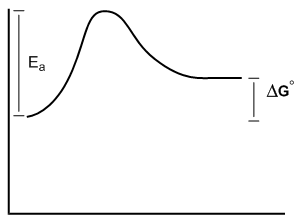## Organic Chemistry

Learn the toughest concepts covered in Organic Chemistry with step-by-step video tutorials and practice problems by world-class tutors.

6. Thermodynamics and Kinetics

# Energy Diagram

You might have heard of the terms thermodynamics and kinetics before, but in chemistry they mean something very specific. Free energy diagrams are going to give us a snapshot of what these terms mean.

1
concept

## Introduction to free energy diagrams.6m
Play a video:

Atoms save energy by forming bonds. Free energy diagrams show overall changes in potential energy during reactions.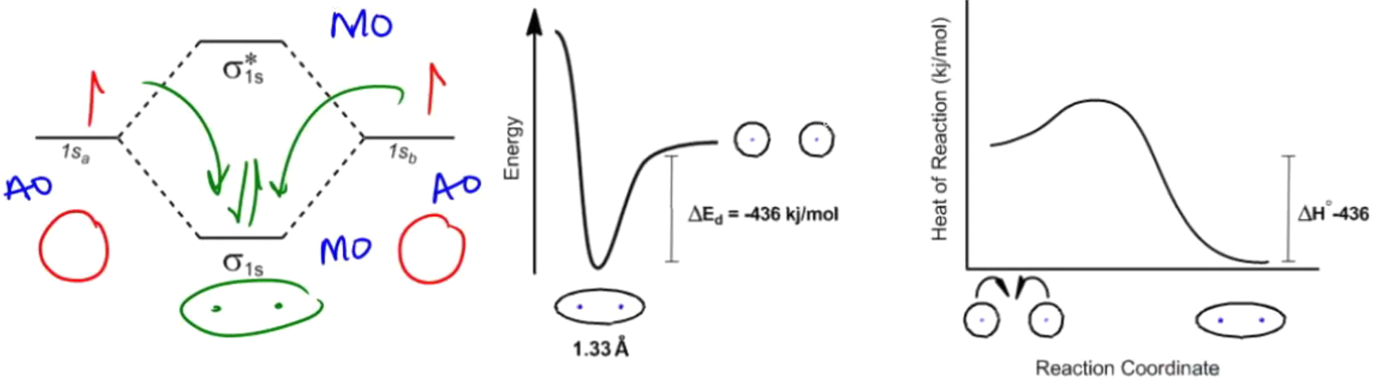Free energy diagrams give us information on the spontaneity and rate of reactions:

• Thermodynamics describes favorability. Determined by Gibbs Free Energy (ΔG° = ΔH° - TΔS).
• Kinetics describes rate. Determined by the activation energy of the rate determining step (Ea).
2
example

## Favorability and rate of Free Energy Diagrams2m
Play a video:
So for this first problem, what we're gonna be looking at right away is if we're gonna be releasing energy or spending energy to make this direction go forward. So we want to do is you wanna look at the energy level of our beginning re agents and then the energy level of our products, and we want to say, Okay, is the energy going down, which means that I'm releasing. Okay, that's also gonna be a negative value of Delta G. Or is the energy going up at the end? Which means I'm spending energy, and that's gonna be a positive value. Okay, so in this case, it's going to be the negative value, right? I'm releasing. Okay. Well, there's actually a word for that. There's a word for when you're releasing energy or when. Overall, you have a negative value of Delta G, and that's called X organic. Okay, so when I say ex organic, that means that I am basically getting some energy back or releasing some energy into the system after the reaction takes place. Okay. Do you guys remember the name of if I'm spending energy? Yeah, that would be undergone IQ. Okay. That means that it's taking. I'm having to put energy into the system to make it happen. Okay, By the way, this line right here just relates to it was supposed to be where it started. Okay, so in this case, this was a negative value, So my spontaneity was negative, which means that this is gonna be X organic. All right, now, in terms of the rate, this one's kind of arbitrary, because I don't have I don't have actual units here or value assigned. What you can see is that I have a few different types of activation energies. I'm gonna have these that are about this big, and then I'm gonna have these down here. That about that big so we can kind of compare them to each other and say this one's gonna be somewhat fast, Okay, because I have a low activation energy. Okay, so it should be what a graph would look like. An A free energy diagram would look like if I had an extra chronic reaction that had a fast rate of reaction. Okay, so now what I wanna do is have you guys do the second one, so go for it.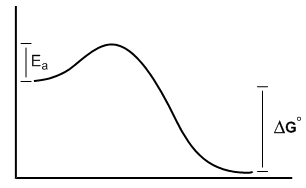3
example

## Favorability and rate of Free Energy Diagrams34s
Play a video:
Now that I've explained how to interpret these graphs thes next problems shouldn't be that bad. So this next one had a positive value of Delta G. So, since it was positive, that means this is an ender gone IQ reaction. I'm gonna have to put energy into the system to make it happen. The activation energy is about the same as before. Okay, So what that means is that this is also reaction That could happen quickly or fast. Okay, so I'm gonna have to put energy into the system, but once I do, it will happen relatively quickly. All right, so let's move on to the next one.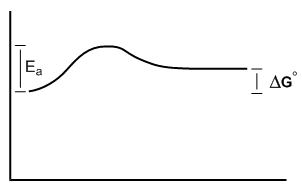4
example

## Favorability and rate of Free Energy Diagrams1m
Play a video:
All right, So this one was a negative value of Delta G. So that means negative equals X organic again. And in this case, you see, the excavation energy got a lot bigger. It's about maybe three times bigger than I drew it before. So what that means is that this was gonna be take much, much longer toe happen, so this would be a slower rate. So it's to be a reaction that is favorable but doesn't actually happen at a very quick, quick pace. Okay, A type of reaction that might fall into this category might be like the burning of wood or whatever. Okay, So, like, wood is when it burns, it gives off carbon dioxide and it gives off, you know, heat and all of that is very, very favorable. That's a very favorable reaction, But does it happen just by itself? Does it just spontaneously burn? No. All right, so there's an example. It's because there's a very high activation energy required in order for combustion to take place. All right, so this would be an example of reaction that's very highly favored, but the activation energy is so high that it doesn't really happen unless we actually overcome the activation energy with heat. Okay. Like actually, like putting some lighter fluid and lighting it on fire. Whatever. All right, so let's go on to the last one.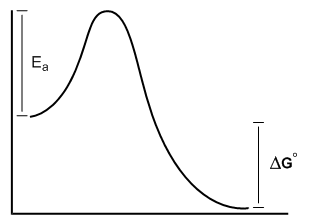5
example

## Favorability and rate of Free Energy Diagrams46s
Play a video: